DraftKings NFC Championship Odds
+225
2.25 to 1Buccaneers
27.0% implied probability

+600
6 to 1Rams
12.5% implied probability

+650
6.5 to 149ers
11.7% implied probability

+750
7.5 to 1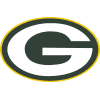Packers
10.3% implied probability

+1100
11 to 1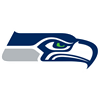Seahawks
7.3% implied probability

+1400
14 to 1Cardinals
5.8% implied probability

+1600
16 to 1Saints
5.2% implied probability

+1800
18 to 1Cowboys
4.6% implied probability

+2500
25 to 1Panthers
3.4% implied probability

+2500
25 to 1Football Team
3.4% implied probability

+3500
35 to 1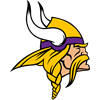Vikings
2.4% implied probability

+3500
35 to 1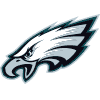Eagles
2.4% implied probability

+5000
50 to 1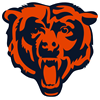Bears
1.7% implied probability

+10000
100 to 1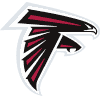Falcons
0.9% implied probability

+10000
100 to 1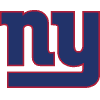Giants
0.9% implied probability

+20000
200 to 1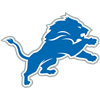Lions
0.4% implied probability